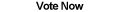Home Articles Components Code Snippets Links

C# Code Snippets# Degrees To Point and Back

Visual C# Kicks

Using simple math we can convert between angles (in degrees) and XY-coordinate points. Useful when working with circle elements.

Platform: .NET Framework 2.0

```/// <summary>
/// Calculates a point that is at an angle from the origin (0 is to the right)
/// </summary>
private PointF DegreesToXY(float degrees, float radius, Point origin)
{
PointF xy = new PointF();
double radians = degrees * Math.PI / 180.0;

return xy;
}

/// <summary>
/// Calculates the angle a point is to the origin (0 is to the right)
/// </summary>
private float XYToDegrees(Point xy, Point origin)
{
int deltaX = origin.X - xy.X;
int deltaY = origin.Y - xy.Y;